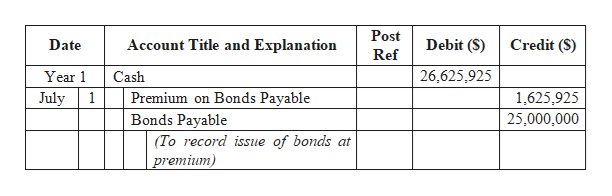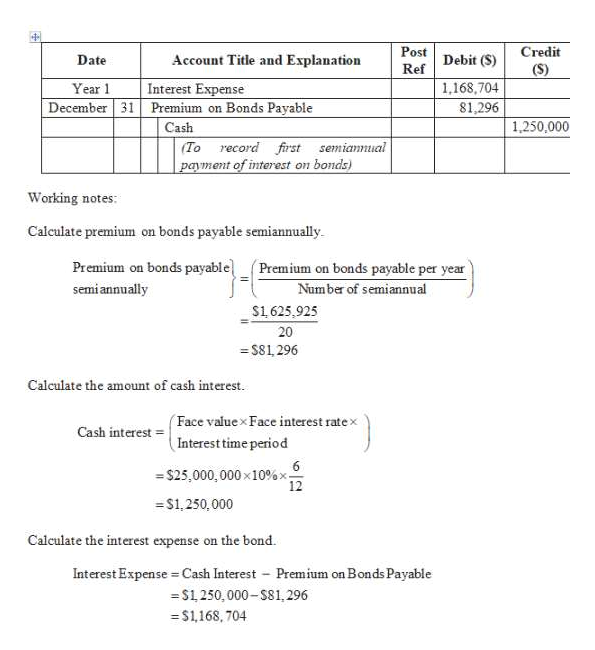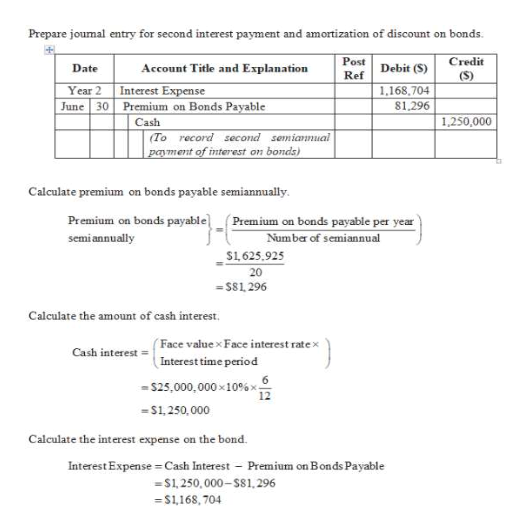# Campbell Inc. produces and sells outdoor equipment. On July 1, Year 1, Campbell Inc. issued \$25,000,000 of 10-year, 10% bonds at a market (effective) interest rate of 9%, receiving cash of \$26,625,925. Interest on the bonds is payable semiannually on December 31 and June 30. The fiscal year of the company is the calendar year.Instructions1. Journalize the entry to record the amount of cash proceeds from the issuance of the bonds on July 1, Year 1.2. Journalize the entries to record the following:a. The first semiannual interest payment on December 31, Year 1, and the amortization of the bond premium, using the straight-line method. Round to the nearest dollar.b. The interest payment on June 30, Year 2, and the amortization of the bond premium, using the straight-line method. Round to the nearest dollar.3. Determine the total interest expense for Year 1.4. Will the bond proceeds always be greater than the face amount of the bonds when the contract rate is greater than the market rate of interest?5. (Appendix 1) Compute the price of \$26,625,925 received for the bonds by using the present value tables in Appendix A at the end of the text. Round to the nearest dollar.

Question
12 views

Campbell Inc. produces and sells outdoor equipment. On July 1, Year 1, Campbell Inc. issued \$25,000,000 of 10-year, 10% bonds at a market (effective) interest rate of 9%, receiving cash of \$26,625,925. Interest on the bonds is payable semiannually on December 31 and June 30. The fiscal year of the company is the calendar year.

Instructions

1. Journalize the entry to record the amount of cash proceeds from the issuance of the bonds on July 1, Year 1.
2. Journalize the entries to record the following:
a. The first semiannual interest payment on December 31, Year 1, and the amortization of the bond premium, using the straight-line method. Round to the nearest dollar.
b. The interest payment on June 30, Year 2, and the amortization of the bond premium, using the straight-line method. Round to the nearest dollar.
3. Determine the total interest expense for Year 1.
4. Will the bond proceeds always be greater than the face amount of the bonds when the contract rate is greater than the market rate of interest?
5. (Appendix 1) Compute the price of \$26,625,925 received for the bonds by using the present value tables in Appendix A at the end of the text. Round to the nearest dollar.

check_circle

Step 1

1.

Journal entry to record the amount of cash proceeds from the issuance of the bonds on July 1, Year 1.help_outlineImage TranscriptionclosePost Account Title and Explanation Debit (\$) Credit (S) Date Ref 26,625,925 Year 1 Cash Premium on Bonds Payable July 1,625,925 Bonds Payable 25,000,000 (To record issue of bonds at premium) fullscreen
Step 2

2.

Journal entry to record first semiannual interest payment and amortization of bond premium on December 31, Year 1.help_outlineImage TranscriptionclosePost Credit Debit (S) Date Account Title and Explanation (S) Ref Year 1 Interest Expense Premium on Bonds Payable 1,168,704 81,296 December 31 1,250,000 Cash (To record first semiannual payment of interest on bonds) Working notes: Calculate premium on bonds payable semiannually. Premium on bonds payable Premium on bonds payable per year Number of semiannual semi annually S1,625,925 20 = \$81, 296 Calculate the amount of cash interest. (Face value x Face interest ratex Interest time period Cash interest = = \$25,000,000 x10%x- 12 =S1,250,000 Calculate the interest expense on the bond. Interest Expense = Cash Interest - Premium on Bonds Payable = S1 250, 000-S81, 296 = \$1,168, 704 fullscreen
Step 3help_outlineImage TranscriptionclosePrepare joumal entry for second interest payment and amortization of discount on bonds. Post Credit Debit (S) Date Account Title and Explanation Ref (S) Year 2 Interest Expense June 30 Premium on Bonds Payable Cash 1,168,704 \$1,296 1,250,000 (To record second semiannual payment of interest on bonds) Calculate premium on bonds payable semiannually. Premium on bonds payable (Premium on bonds payable per year Number of semiannual semi annually S1,625,925 20 = \$81, 296 Calculate the amount of cash interest. ( Face value x Face interest ratex Interest time period -\$25,000,000 x10%x Cash interest = 12 =S1,250,000 Calculate the interest expense on the bond. Interest Expense = Cash Interest - Premium on Bonds Payable =\$1, 250,000- S81,296 = \$1,168, 704 fullscreen

### Want to see the full answer?

See Solution

#### Want to see this answer and more?

Solutions are written by subject experts who are available 24/7. Questions are typically answered within 1 hour.*

See Solution
*Response times may vary by subject and question.
Tagged in